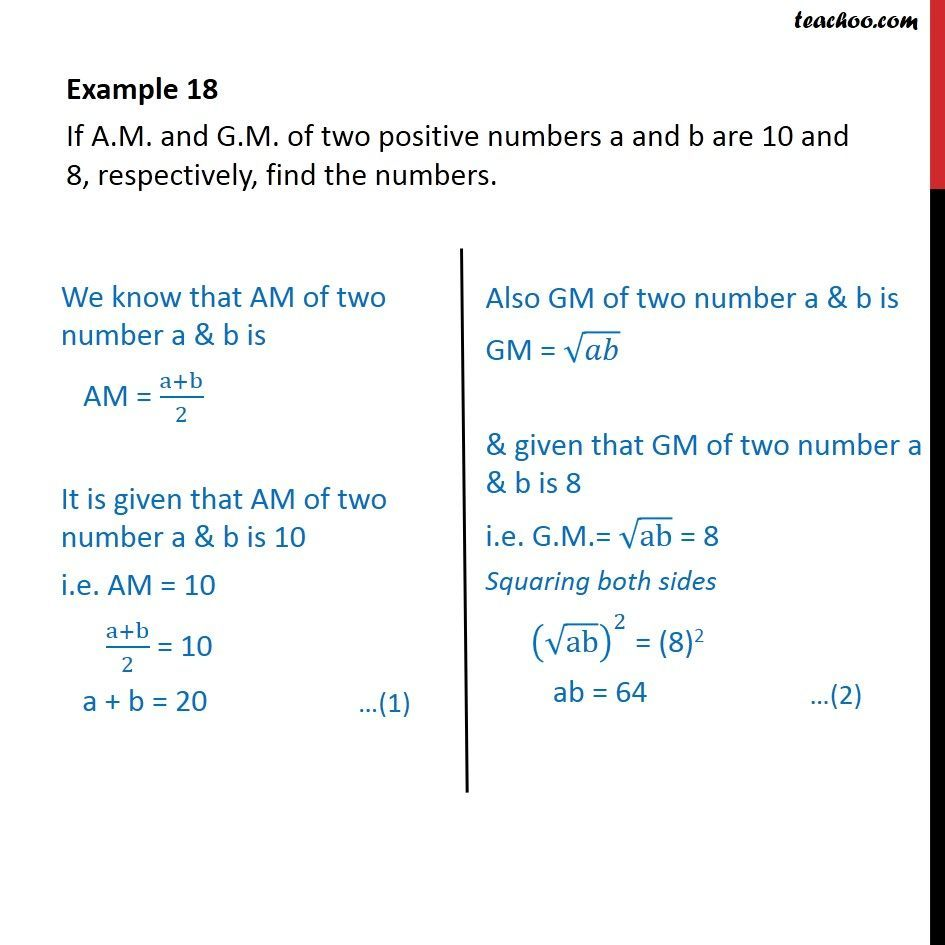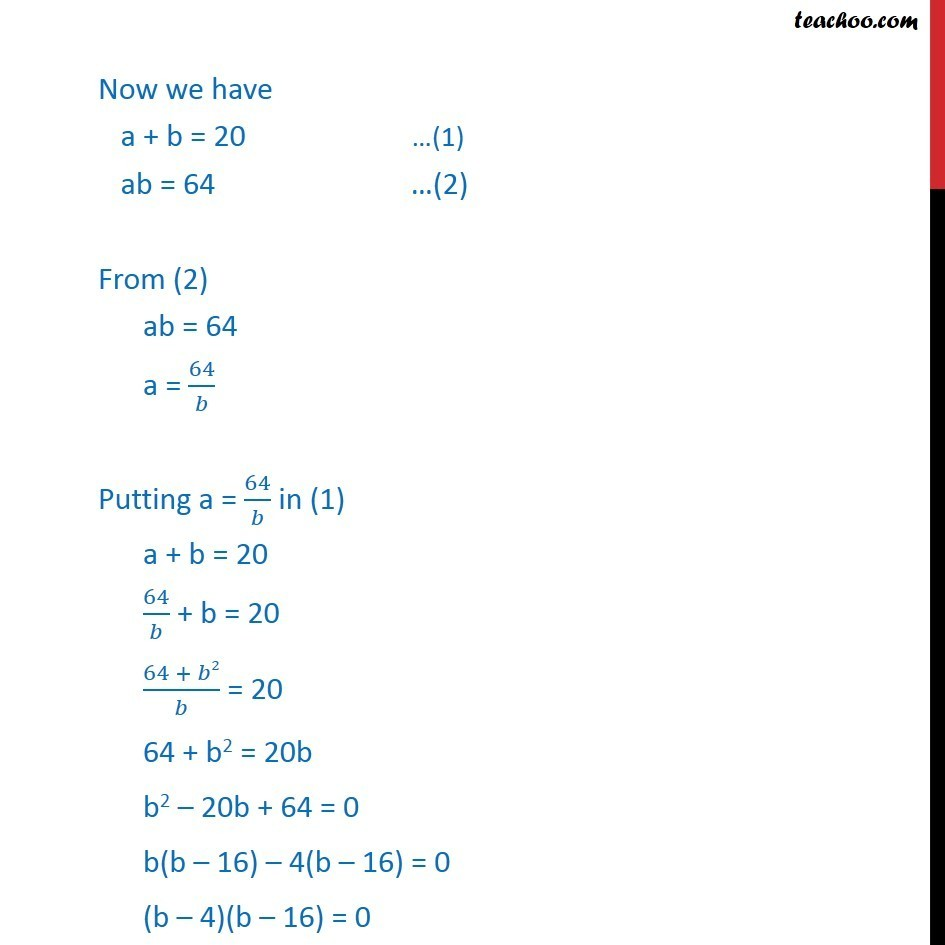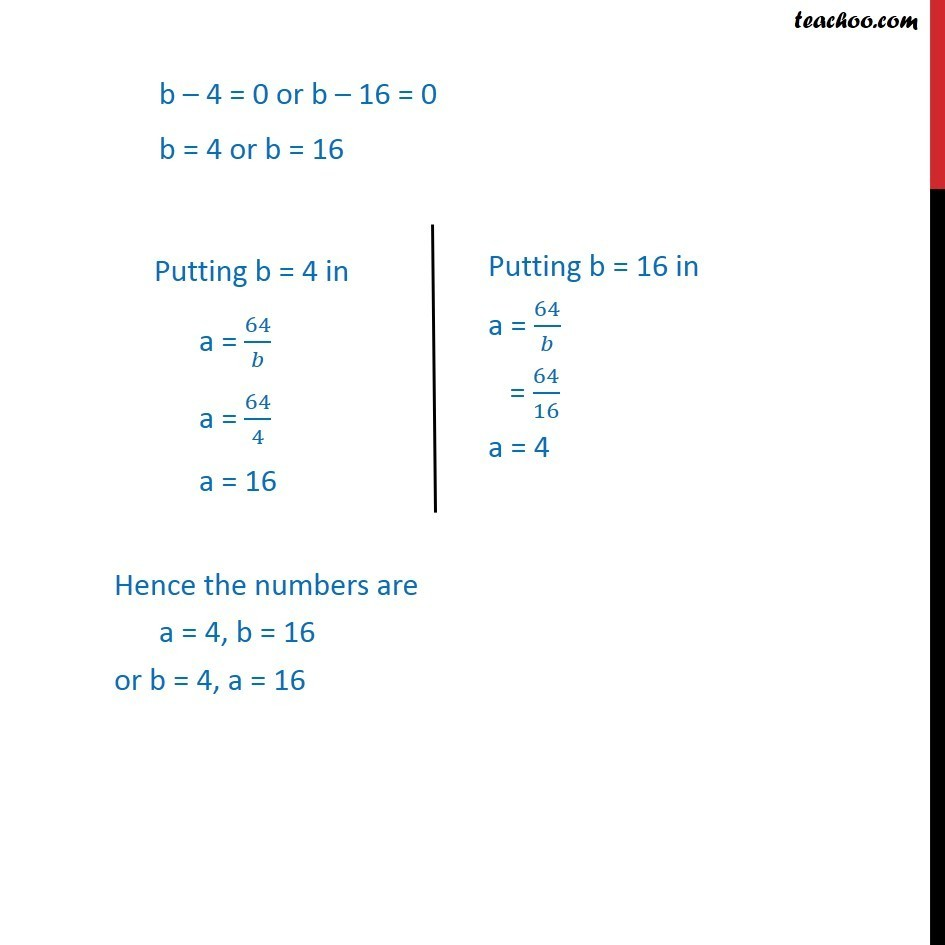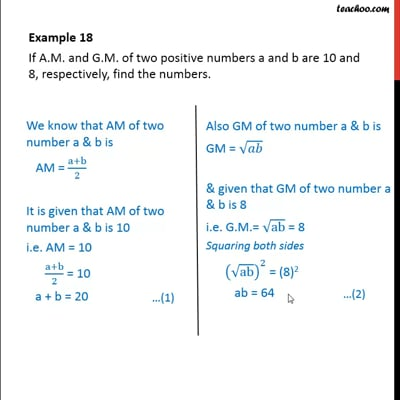AM and GM (Arithmetic Mean And Geometric mean)

Chapter 9 Class 11 Sequences and Series
Concept wiseThis video is only available for Teachoo black users

Introducing your new favourite teacher - Teachoo Black, at only ₹83 per month

### Transcript

Example 18 If A.M. and G.M. of two positive numbers a and b are 10 and 8, respectively, find the numbers. Now we have a + b = 20 (1) ab = 64 (2) From (2) ab = 64 a = 64/ Putting a = 64/ in (1) a + b = 20 64/ + b = 20 (64 + 2)/ = 20 64 + b2 = 20b b2 20b + 64 = 0 b(b 16) 4(b 16) = 0 (b 4)(b 16) = 0 b 4 = 0 or b 16 = 0 b = 4 or b = 16 Hence the numbers are a = 4, b = 16 or b = 4, a = 16